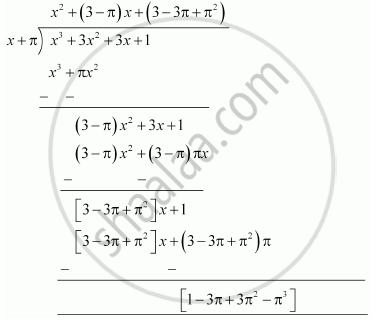Share

# Find the remainder when x^3 + 3x^2 + 3x + 1 is divided by x + π. - CBSE Class 9 - Mathematics

#### Question

Find the remainder when x3 + 3x2 + 3x + 1 is divided by x + π.

#### Solution 1

Let p(x) = x3 + 3x2 + 3x + 1

x + π = 0 ⇒ x = -π

∴ Remainder = (-π)3 + 3(-π)2 - 3(-π) + 1

= -π3 + 3π2 - 3π + 1

Therefore, the remainder is -π3 + 3π2 - 3π + 1.

#### Solution 2

By long division,Therefore, the remainder is -π3 + 3π2 - 3π + 1.

Is there an error in this question or solution?

#### APPEARS IN

Solution Find the remainder when x^3 + 3x^2 + 3x + 1 is divided by x + π. Concept: Remainder Theorem.
S